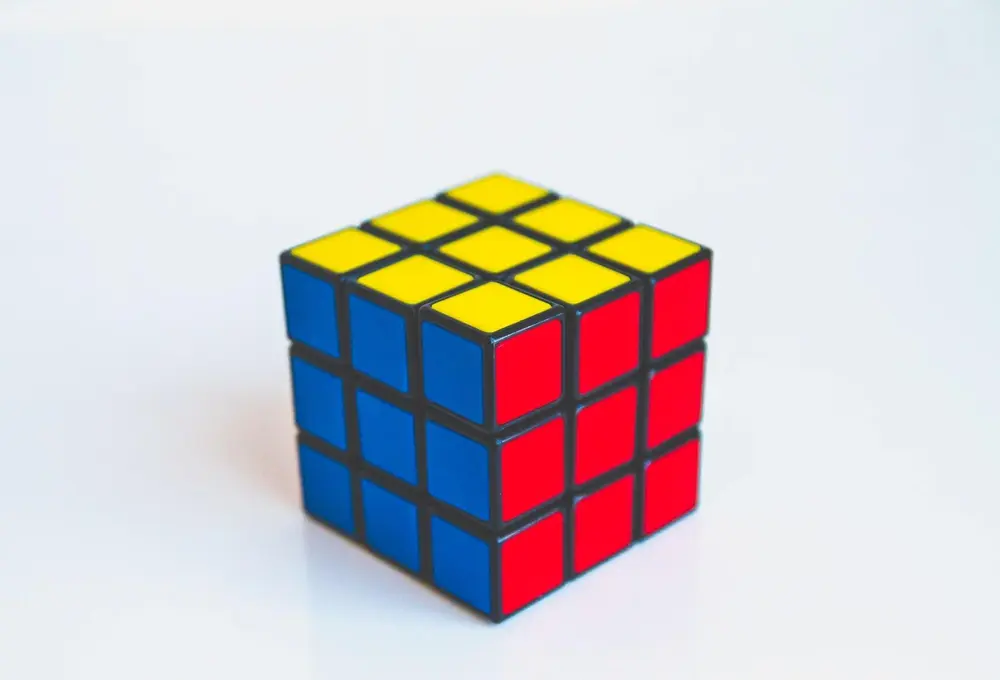# Similar Triangles

If two shapes are similar, one is an enlargement of the another. This means that the two shapes will have the same angles and their sides will be in the same proportion (e.g. the sides of one triangle will all be 3 times the sides of the other etc.).

• angle A = angle D
• angle B = angle E
• angle C = angle F

AB/DE = BC/EF = AC/DF = perimeter of ABC/ perimeter of DEF

Two triangles are similar if:

• 1) 3 angles of 1 triangle are the same as 3 angles of the other
• or 2) 3 pairs of corresponding sides are in the same ratio
• or 3) An angle of 1 triangle is the same as the angle of the other triangle and the sides containing these angles are in the same ratio.

Example:

• In the above diagram, the triangles are similar. EF = 6cm and BC = 2cm . What is the length of DE if AB is 3cm?
• EF = 3BC, so DE = 3AB = 9cm.
Shape and Space

Shape and Space

Shape and Space

Shape and Space

Shape and Space

Shape and Space